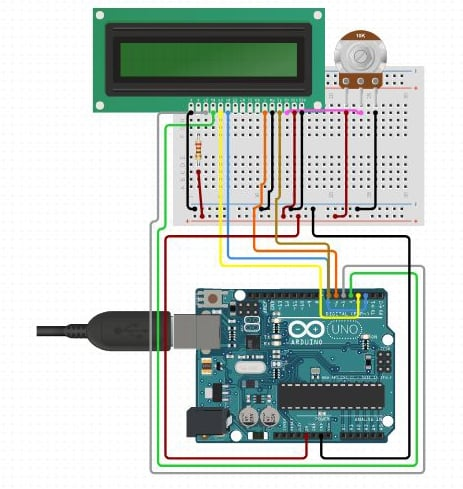The main principle behind this is the voltage divider network.

Summary

The main principle behind this is the voltage divider network. The value of the unknown resistance is displayed on the 16*2 LCD display.

The working of this Resistance Meter is very simple and can be described using a voltage divider network shown below.

From the voltage divider network of resistors R1 and R2,

Vout = Vin * R2 / ( R1+R2 )

From the above equation, we can deduce the value of R2 as

R2 = Vout * R1 / (Vin - Vout)

Where R1 = known resistance

R2 = Unknown resistance

Vout = voltage at R2 with respect to ground

Vin = voltage presented at the 5V pin of Arduino

So if we see the value of voltage across unknown resistance (Vout), we can clearly determine the unknown resistance R2. Here we have read the voltage value Vout using the analog pin A0 and converted those digital values (0 -1023) into voltage as explained in the Code.

If the value of the known Resistance is higher or smaller than the unknown resistance the error will be more. So it is necessary to keep the known resistance value next to the unknown resistance.```        ```#include<LiquidCrystal.h>

LiquidCrystal lcd(2,3,4,5,6,7);   //rs,e,d4,d5,d6,d7

int Vin=5;        //voltage at 5V pin of arduino
float Vout=0;     //voltage at A0 pin of arduino
float R1=3300;    //value of known resistance
float R2=0;       //value of unknown resistance
int a2d_data=0;
float buffer=0;

void setup()
{
lcd.begin(16,2);
}

void loop()
{
if(a2d_data)
{
buffer=a2d_data*Vin;
Vout=(buffer)/1024.0;
buffer=Vout/(Vin-Vout);
R2=R1*buffer;

lcd.setCursor(4,0);
lcd.print("ohm meter");

lcd.setCursor(0,1);
lcd.print("R (ohm) = ");
lcd.print(R2);

delay(1000);
}
}```
```